# Plotting Data on Google Map using Python’s pygmaps package

• Last Updated : 15 Oct, 2020

pygmaps is a matplotlib-like interface to generate the HTML and javascript to render all the data users would like on top of Google Maps.

Command to install pygmaps :

```pip install pygmaps (on windows)
sudo pip3 install pygmaps (on linix / unix)
```

Code #1 : To create a Base Map.

 `# import required package``import` `pygmaps`` ` `# maps method return map object``# 1st argument is center latitude``# 2nd argument is center longitude``# 3ed argument zoom level``mymap1 ``=` `pygmaps.maps(``30.3164945``, ``78.03219179999999``, ``15``)`` ` `# create the HTML file which includes``# google map. Pass the absolute path``# as an argument.``mymap1.draw(``'pygmap1.html'``)`

Output: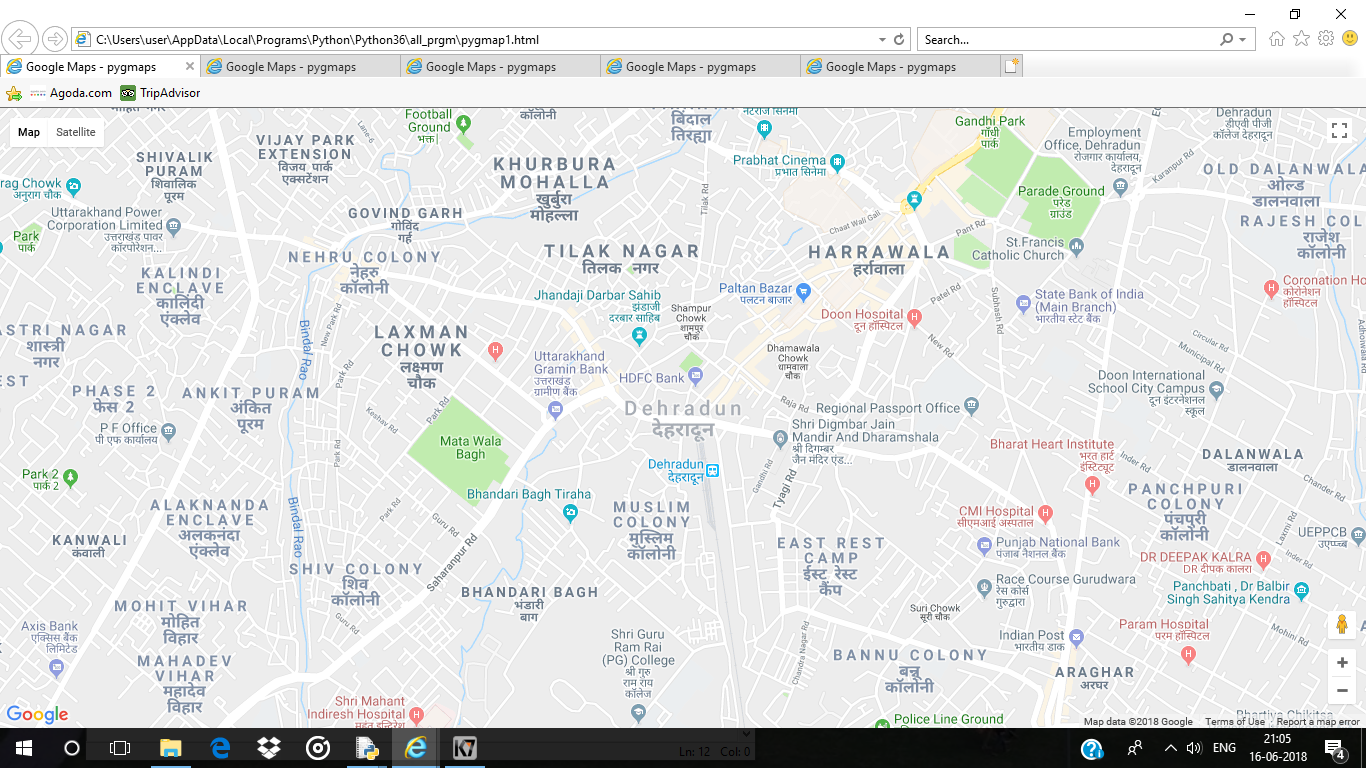Code #2 : To draw grids on map

 `# importing pygmaps``import` `pygmaps`` ` `mymap2 ``=` `pygmaps.maps(``30.3164945``, ``78.03219179999999``, ``15``)`` ` `# draw grids on the map``# 1st argument is the starting point of latitude``# 2nd argument is the ending point of latitude``# 3rd argument is grid size in latitude``# 4th argument is the starting point of longitude``# 5th argument is the ending point of longitude``# 6th argument is grid size in longitude``mymap2.setgrids(``30.31``, ``30.32``, ``0.001``,``                ``78.04``, ``78.03``, ``0.001``)``                 ` `mymap2.draw(``'pygmap2.html'``)`

Output: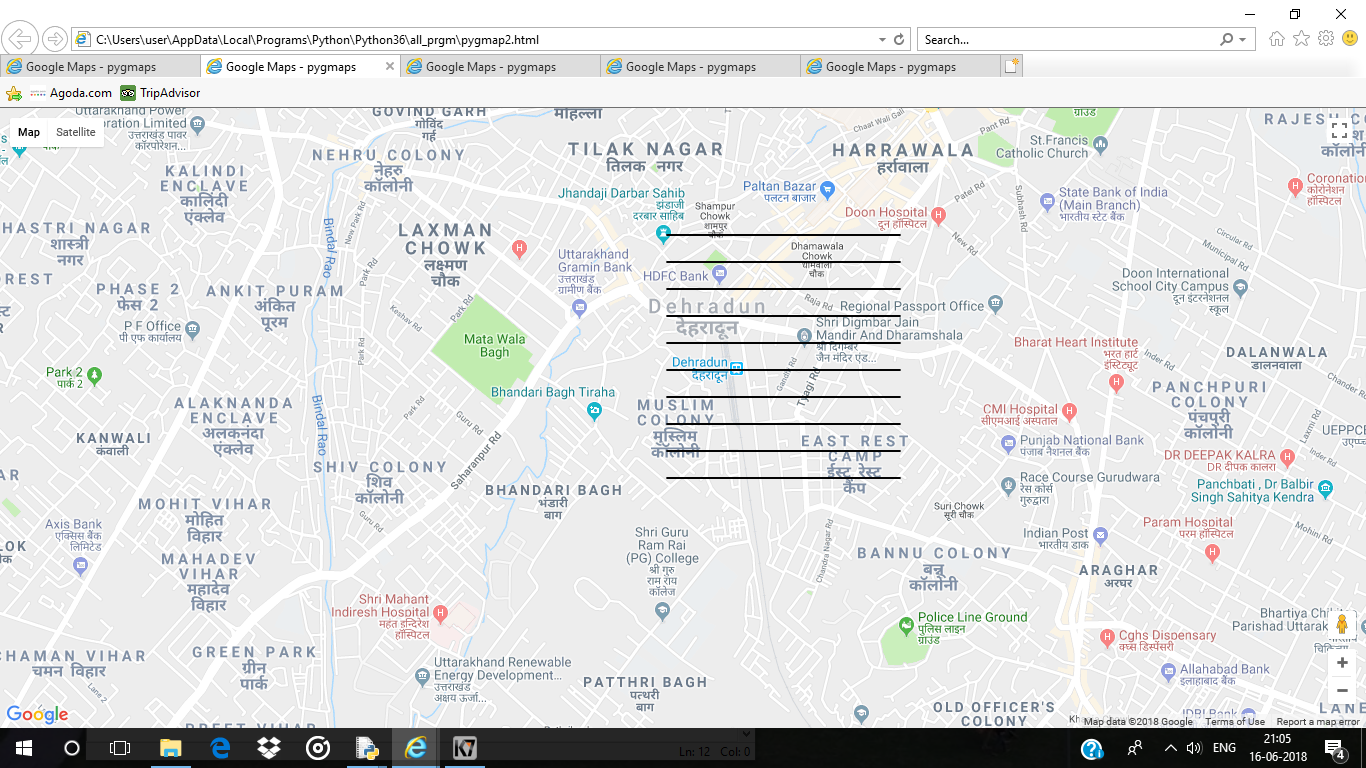Code #3 : To add a point into a map

 `# importing pygmaps``import` `pygmaps`` ` `# list of latitudes``latitude_list ``=` `[``30.3358376``, ``30.307977``, ``30.3216419``,``                 ``30.3427904``, ``30.378598``, ``30.3548185``,``                 ``30.3345816``, ``30.387299``, ``30.3272198``,``                 ``30.3840597``, ``30.4158``, ``30.340426``,``                 ``30.3984348``, ``30.3431313``, ``30.273471``]`` ` `# list of longitudes``longitude_list ``=` `[``77.8701919``, ``78.048457``, ``78.0413095``,``                  ``77.886958``, ``77.825396``, ``77.8460573``,``                  ``78.0537813``, ``78.090614``, ``78.0355272``,``                  ``77.9311923``, ``77.9663``, ``77.952092``,``                  ``78.0747887``, ``77.9555512``, ``77.9997158``]`` ` `mymap3 ``=` `pygmaps.maps(``30.3164945``, ``78.03219179999999``, ``15``)`` ` ` ` `for` `i ``in` `range``(``len``(latitude_list)):`` ` `    ``# add a point into a map``    ``# 1st argument is latitude``    ``# 2nd argument is longitude``    ``# 3rd argument is colour of the point showed in thed map``    ``# using HTML colour code e.g.``    ``# red "# FF0000", Blue "# 0000FF", Green "# 00FF00"``    ``mymap3.addpoint(latitude_list[i], longitude_list[i], ``"# FF0000"``)``     ` `mymap3.draw(``'pygmap3.html'``)`

Output: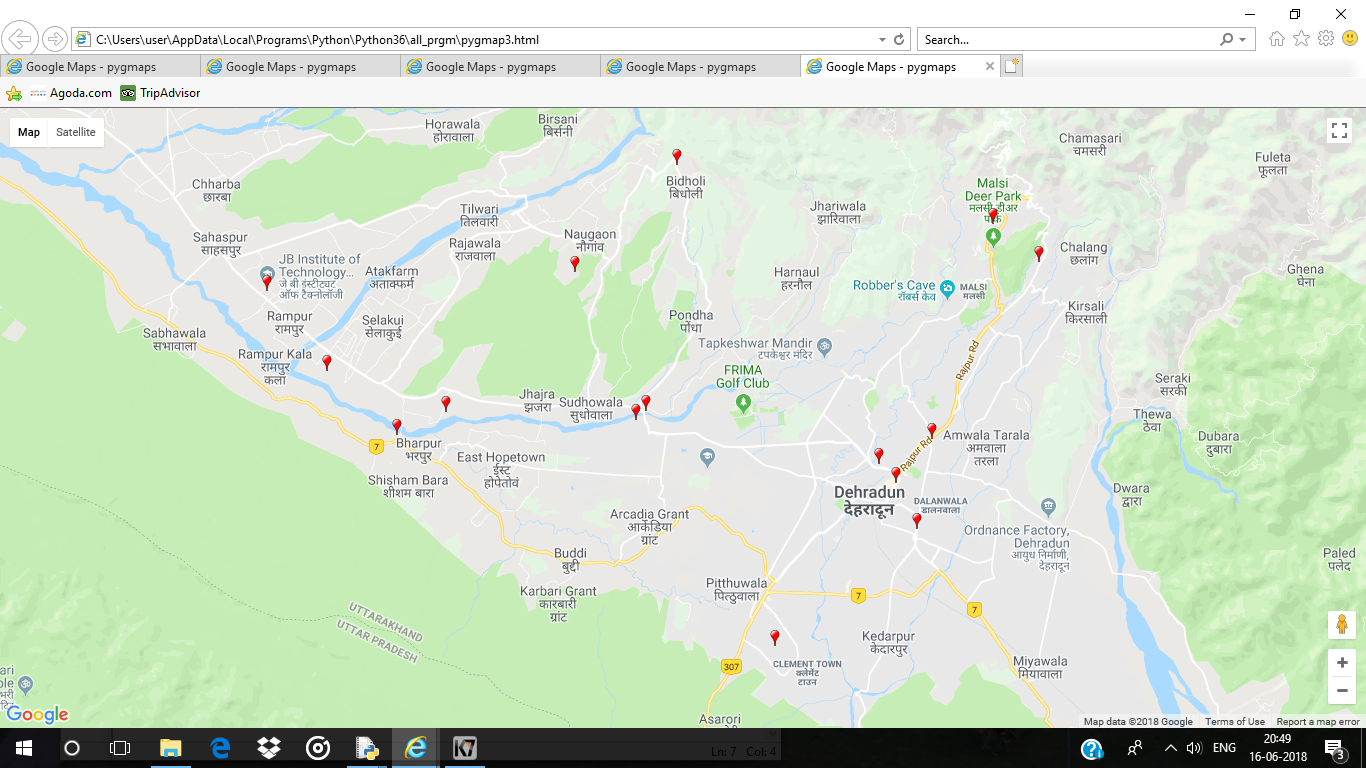Code #4 : To Draw a circle of given radius

 `import` `pygmaps`` ` `mymap4 ``=` `pygmaps.maps(``30.3164945``, ``78.03219179999999``, ``15``)`` ` `# Draw a circle of given radius``# 1st argument is latitude``# 2nd argument is longitude``# 3rd argument is radius (in meter)``# 4th argument is colour of the circle``mymap4.addradpoint(``30.307977``, ``78.048457``, ``95``, ``"# FF0000"``)``mymap4.draw(``'pygmap4.html'``)`

Output :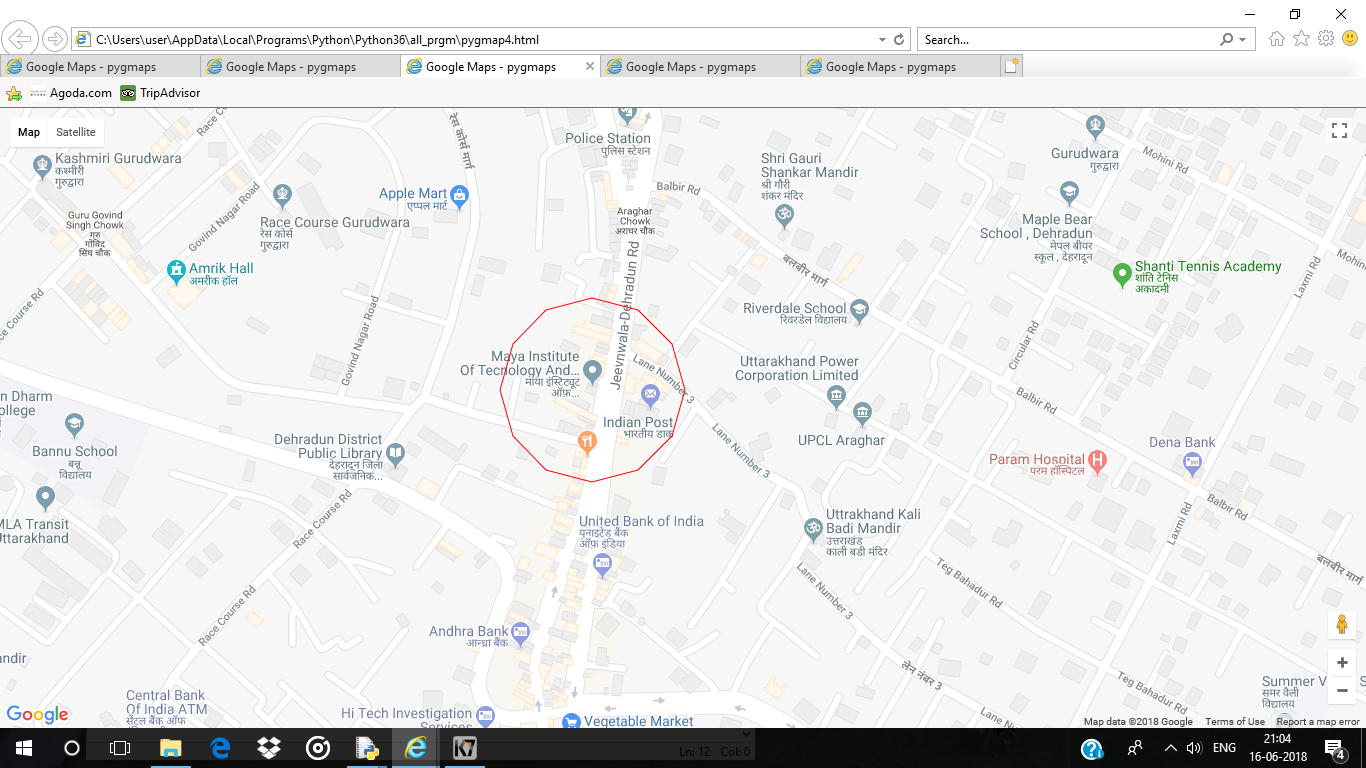Code #5 : To draw a line in b/w the given coordinates

 `# Importing pygmaps``import` `pygmaps`` ` `mymap5 ``=` `pygmaps.maps(``30.3164945``, ``78.03219179999999``, ``15``)`` ` `latitude_list ``=``[``30.343769``, ``30.307977``]``longitude_list ``=``[``77.999559``, ``78.048457``]`` ` `for` `i ``in` `range``(``len``(latitude_list)) :``    ``mymap5.addpoint(latitude_list[i], longitude_list[i], ``"# FF0000"``)`` ` `# list of coordinates         ``path ``=` `[(``30.343769``, ``77.999559``),``        ``(``30.307977``, ``78.048457``)]`` ` `# draw a line in b / w the given coordinates``# 1st argument is list of coordinates``# 2nd argument is colour of the line``mymap5.addpath(path, ``"# 00FF00"``)`` ` `mymap5.draw(``'pygmap5.html'``)`

Output: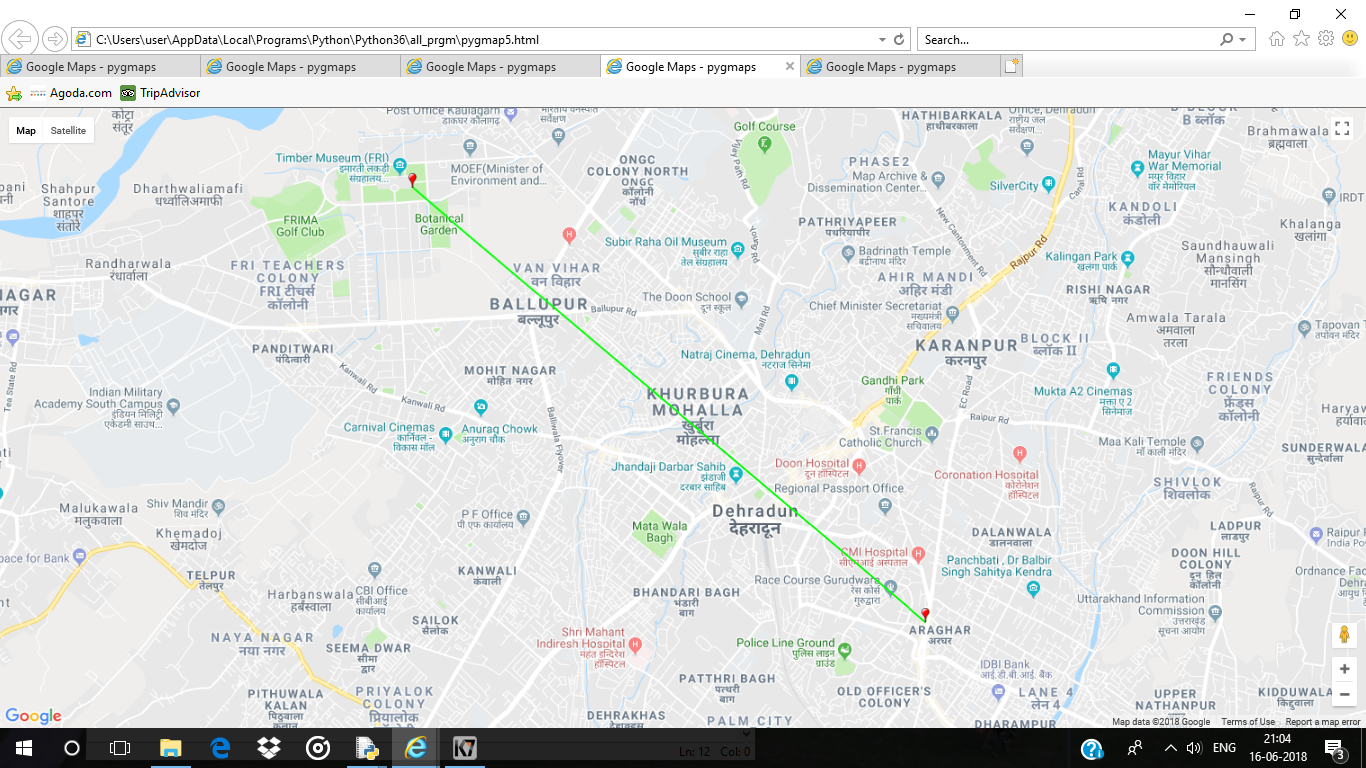My Personal Notes arrow_drop_up